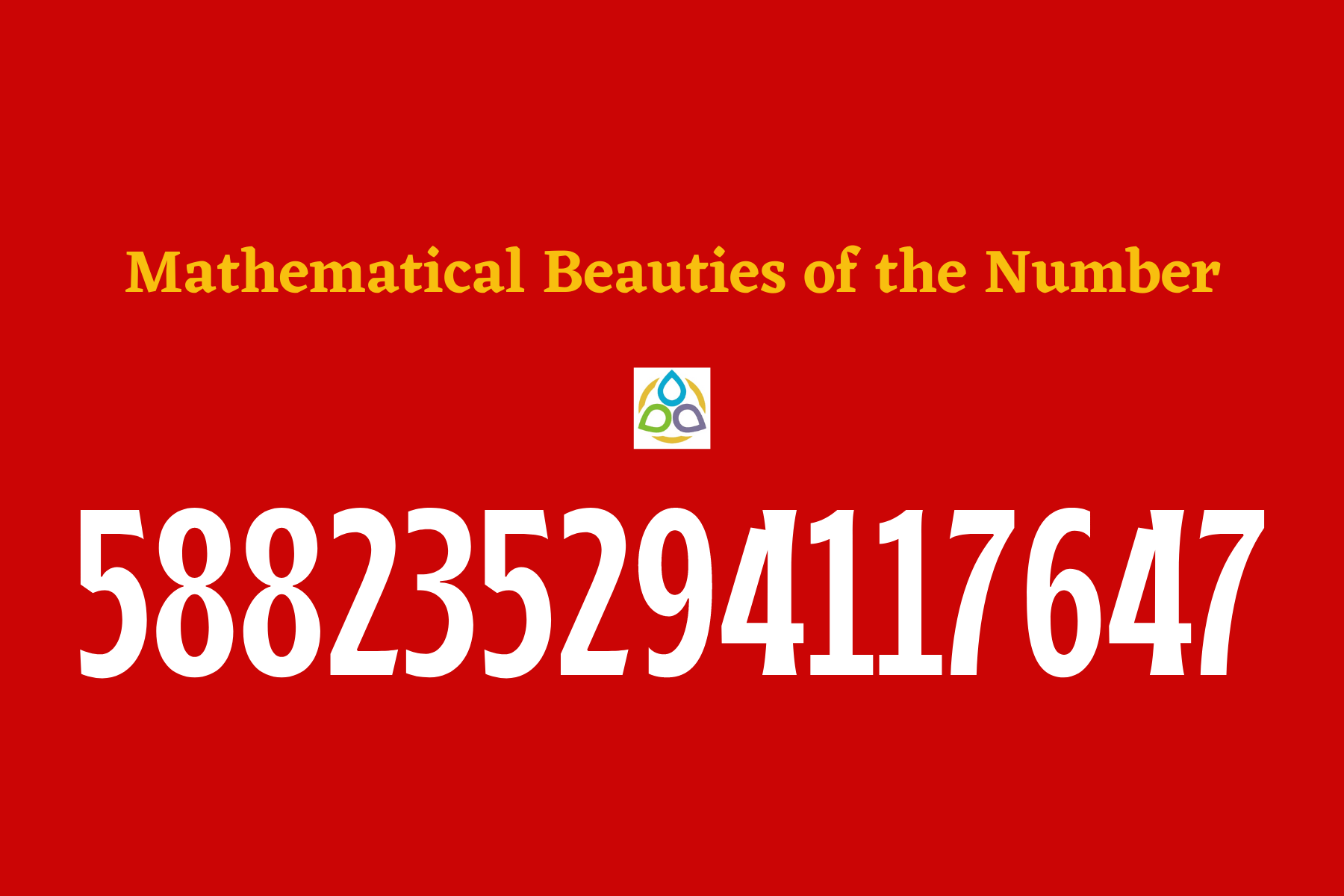# Mathematical Beauties of the Number 588235294117647

The mathematician’s patterns, like the painter’s or the poet’s, must be beautiful; the ideas, like the colours or the words, must fit together in a harmonious way. Beauty is the first test: there is no permanent place in the world for ugly mathematics.
G. H. Hardy

Greetings to all visitors of Math1089 – Mathematics for All.

I’m thrilled you’re here. I wanted to express my gratitude for your valuable time spent on Math1089. Your visit means a lot to me and I truly appreciate you taking the time from your hectic schedule to check out this blog.

Let’s delve into the beauty of the number 588235294117647! This lovely number can be found in the fraction 1/17. We’ll begin by exploring Midy’s theorem and uncovering other intriguing characteristics of this number.

Midy’s Theorem If the period of a repeating decimal for a/p, where p is prime and a/p is a reduced fraction, has an even number of digits, then dividing the repeating portion into halves and adding gives a string of 9s. In the case of 1/17 (oneseventeenth), the period is 16. In fact, we have

When we multiply 588235294117647 by other numbers, notice how it yields numbers in the same order but with a different starting point. In fact, the first digit of every product goes to the end of the number to form the next product. Otherwise, the order of the digits is intact. Moreover, the sum of the digits in each of these product numbers is equal to 72.

The product of the number 0588235294117647 and 17 generates the following result:

0588235294117647 × 17    = 9999999999999999

The product of the number 0588235294117647 and multiples of 17 generates the following set of results:

A few noteworthy observations are as follows:

(a) With an increasing multiplier of 0588235294117647, the leftmost digits of the product will be in ascending order, such as 1, 2, 3, and so on.

(b) As the multiplier of 0588235294117647 increases, the rightmost digits of the product will be in decreasing order, such as 8, 7, 6, and so on.

(c) When the sum of the multipliers is held constant (equal to 187), the products are reverse of each other.

0588235294117647 × 34    = 19999999999999998

0588235294117647 × 153 = 89999999999999991

0588235294117647 × 51    = 29999999999999997

0588235294117647 × 136 = 79999999999999992

0588235294117647 × 68    = 39999999999999996

0588235294117647 × 119 = 69999999999999993

0588235294117647 × 85    = 49999999999999995

0588235294117647 × 102 = 59999999999999994

## The sum of the digits of the number

We can write the sum of the digits of the number 0588235294117647 in different ways to get different results. Here are a few examples:

(a) The sum of all the digits is

0 + 5 + 8 + 8 + 2 + 3 + 5 + 2 + 9 + 4 + 1 + 1 + 7 + 6 + 4 + 7

= 72

≡ 0 (mod 9).

In other words, it is divisible by 9.

(b) The sum of the digits taken two at a time is

05 + 88 + 23 + 52 + 94 + 11 + 76 + 47

= 396

≡ 0 (mod 99).

In other words, it is divisible by 99.

(c) The sum of the digits taken four at a time is

0588 + 2352 + 9411 + 7647

= 19998

≡ 0 (mod 9999).

In other words, it is divisible by 9999.

(d) The sum of all the digits taken eight at a time is

05882352 + 94117647

= 99999999

≡ 0 (mod 99999999).

In other words, it is divisible by 99999999.

We can show that 16 is the smallest value of n such that 10n – 1 is divisible by 17. In that case, (1016 – 1) / 17 = 0588235294117647 (16 digits) and we get

We welcome your ideas with open arms and reverence! Looking forward to seeing you soon on ”Math1089 – Mathematics for All” for another fascinating mathematics blog.